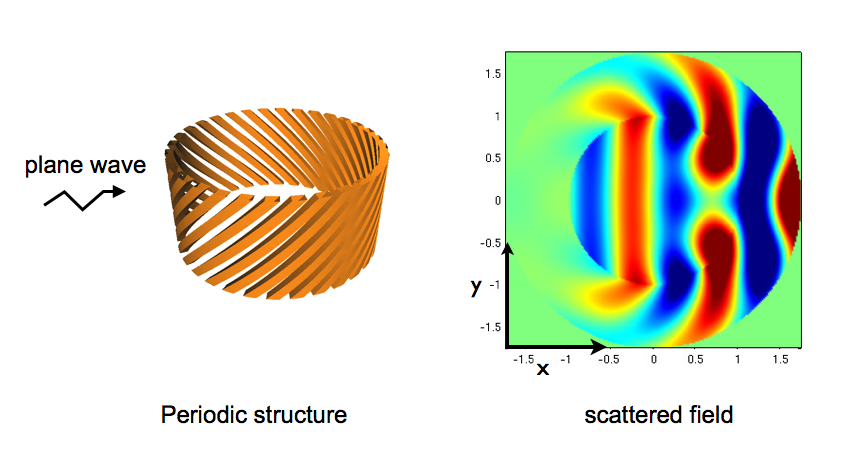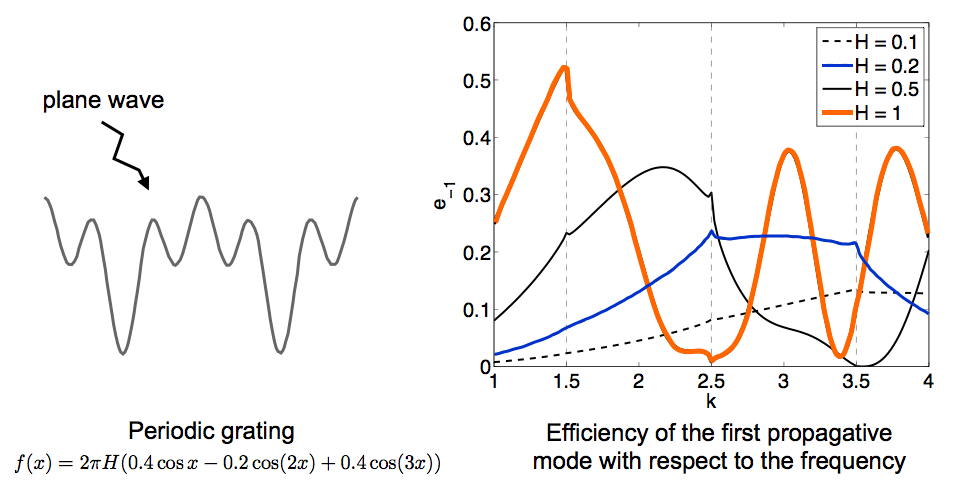## Research area

My work is dedicated to the mathematical study of periodic structures. I particularly focus on the following points:

### Construction and analysis of approximate model for periodic structures

• Asymptotic Analysis (periodic homogenization, asymptotic expansion)
• Maxwell equations, Helmoltz equation
• Numerical simulations### Simulation of the scattering from periodic gratings using integral equations

• Development of a robust numerical method valid at and around Wood anomalies
• Periodic Green function
• Integral equations### Mathematical and numerical investigation of periodic waveguides

• Localized and guided modes
• periodic graphs
• Spectral theory